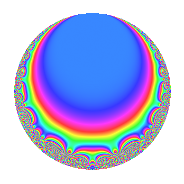Properties

 Label 23.3.b.aLevel 23 Weight 3 Character orbit 23.b Self dual Yes Analytic conductor 0.627 Analytic rank 0 Dimension 3 CM disc. -23 Inner twists 2

Related objects

Newspace parameters

 Level: $$N$$ = $$23$$ Weight: $$k$$ = $$3$$ Character orbit: $$[\chi]$$ = 23.b (of order $$2$$ and degree $$1$$)

Newform invariants

 Self dual: Yes Analytic conductor: $$0.626704608029$$ Analytic rank: $$0$$ Dimension: $$3$$ Coefficient field: 3.3.621.1 Coefficient ring: $$\Z[a_1, a_2, a_3]$$ Coefficient ring index: $$1$$ Sato-Tate group: $\mathrm{U}(1)[D_{2}]$

$q$-expansion

Coefficients of the $$q$$-expansion are expressed in terms of a basis $$1,\beta_1,\beta_2$$ for the coefficient ring described below. We also show the integral $$q$$-expansion of the trace form.

 $$f(q)$$ $$=$$ $$q$$ $$+ ( \beta_{1} + \beta_{2} ) q^{2}$$ $$+ ( -2 \beta_{1} - \beta_{2} ) q^{3}$$ $$+ ( 4 + \beta_{1} - 2 \beta_{2} ) q^{4}$$ $$+ ( -11 - 3 \beta_{1} + 2 \beta_{2} ) q^{6}$$ $$+ ( -7 + 4 \beta_{1} + 4 \beta_{2} ) q^{8}$$ $$+ ( 9 + 6 \beta_{1} - \beta_{2} ) q^{9}$$ $$+O(q^{10})$$ $$q$$ $$+ ( \beta_{1} + \beta_{2} ) q^{2}$$ $$+ ( -2 \beta_{1} - \beta_{2} ) q^{3}$$ $$+ ( 4 + \beta_{1} - 2 \beta_{2} ) q^{4}$$ $$+ ( -11 - 3 \beta_{1} + 2 \beta_{2} ) q^{6}$$ $$+ ( -7 + 4 \beta_{1} + 4 \beta_{2} ) q^{8}$$ $$+ ( 9 + 6 \beta_{1} - \beta_{2} ) q^{9}$$ $$+ ( 1 - 11 \beta_{1} - 11 \beta_{2} ) q^{12}$$ $$+ ( -2 \beta_{1} + 7 \beta_{2} ) q^{13}$$ $$+ ( 16 - 7 \beta_{1} - 7 \beta_{2} ) q^{16}$$ $$+ ( 13 + 22 \beta_{1} + 11 \beta_{2} ) q^{18}$$ $$-23 q^{23}$$ $$+ ( -44 + 2 \beta_{1} + 15 \beta_{2} ) q^{24}$$ $$+ 25 q^{25}$$ $$+ ( 29 - 11 \beta_{1} - 14 \beta_{2} ) q^{26}$$ $$+ ( -38 - 18 \beta_{1} - 9 \beta_{2} ) q^{27}$$ $$+ ( -2 \beta_{1} - 17 \beta_{2} ) q^{29}$$ $$+ ( 22 \beta_{1} + 7 \beta_{2} ) q^{31}$$ $$+ ( -28 - 7 \beta_{1} + 14 \beta_{2} ) q^{32}$$ $$+ ( 85 + 22 \beta_{1} - 5 \beta_{2} ) q^{36}$$ $$+ ( -14 + 6 \beta_{1} + 23 \beta_{2} ) q^{39}$$ $$+ ( -26 \beta_{1} + 7 \beta_{2} ) q^{41}$$ $$+ ( -23 \beta_{1} - 23 \beta_{2} ) q^{46}$$ $$+ ( 22 \beta_{1} - 17 \beta_{2} ) q^{47}$$ $$+ ( 77 - 11 \beta_{1} - 30 \beta_{2} ) q^{48}$$ $$+ 49 q^{49}$$ $$+ ( 25 \beta_{1} + 25 \beta_{2} ) q^{50}$$ $$+ ( -103 + 29 \beta_{1} + 29 \beta_{2} ) q^{52}$$ $$+ ( -99 - 65 \beta_{1} - 20 \beta_{2} ) q^{54}$$ $$+ ( -91 + 13 \beta_{1} + 34 \beta_{2} ) q^{58}$$ $$+ 26 q^{59}$$ $$+ ( 101 + 37 \beta_{1} - 14 \beta_{2} ) q^{62}$$ $$+ ( -15 - 28 \beta_{1} - 28 \beta_{2} ) q^{64}$$ $$+ ( 46 \beta_{1} + 23 \beta_{2} ) q^{69}$$ $$+ ( -26 \beta_{1} + 31 \beta_{2} ) q^{71}$$ $$+ ( -11 + 46 \beta_{1} + 51 \beta_{2} ) q^{72}$$ $$+ ( -26 \beta_{1} - 41 \beta_{2} ) q^{73}$$ $$+ ( -50 \beta_{1} - 25 \beta_{2} ) q^{75}$$ $$+ ( 133 - 25 \beta_{1} - 60 \beta_{2} ) q^{78}$$ $$+ ( 81 + 76 \beta_{1} + 38 \beta_{2} ) q^{81}$$ $$+ ( -43 - 59 \beta_{1} - 14 \beta_{2} ) q^{82}$$ $$+ ( 82 + 6 \beta_{1} - 49 \beta_{2} ) q^{87}$$ $$+ ( -92 - 23 \beta_{1} + 46 \beta_{2} ) q^{92}$$ $$+ ( -182 - 66 \beta_{1} - \beta_{2} ) q^{93}$$ $$+ ( -19 + 61 \beta_{1} + 34 \beta_{2} ) q^{94}$$ $$+ ( -7 + 77 \beta_{1} + 77 \beta_{2} ) q^{96}$$ $$+ ( 49 \beta_{1} + 49 \beta_{2} ) q^{98}$$ $$+O(q^{100})$$ $$\operatorname{Tr}(f)(q)$$ $$=$$ $$3q$$ $$\mathstrut +\mathstrut 12q^{4}$$ $$\mathstrut -\mathstrut 33q^{6}$$ $$\mathstrut -\mathstrut 21q^{8}$$ $$\mathstrut +\mathstrut 27q^{9}$$ $$\mathstrut +\mathstrut O(q^{10})$$ $$3q$$ $$\mathstrut +\mathstrut 12q^{4}$$ $$\mathstrut -\mathstrut 33q^{6}$$ $$\mathstrut -\mathstrut 21q^{8}$$ $$\mathstrut +\mathstrut 27q^{9}$$ $$\mathstrut +\mathstrut 3q^{12}$$ $$\mathstrut +\mathstrut 48q^{16}$$ $$\mathstrut +\mathstrut 39q^{18}$$ $$\mathstrut -\mathstrut 69q^{23}$$ $$\mathstrut -\mathstrut 132q^{24}$$ $$\mathstrut +\mathstrut 75q^{25}$$ $$\mathstrut +\mathstrut 87q^{26}$$ $$\mathstrut -\mathstrut 114q^{27}$$ $$\mathstrut -\mathstrut 84q^{32}$$ $$\mathstrut +\mathstrut 255q^{36}$$ $$\mathstrut -\mathstrut 42q^{39}$$ $$\mathstrut +\mathstrut 231q^{48}$$ $$\mathstrut +\mathstrut 147q^{49}$$ $$\mathstrut -\mathstrut 309q^{52}$$ $$\mathstrut -\mathstrut 297q^{54}$$ $$\mathstrut -\mathstrut 273q^{58}$$ $$\mathstrut +\mathstrut 78q^{59}$$ $$\mathstrut +\mathstrut 303q^{62}$$ $$\mathstrut -\mathstrut 45q^{64}$$ $$\mathstrut -\mathstrut 33q^{72}$$ $$\mathstrut +\mathstrut 399q^{78}$$ $$\mathstrut +\mathstrut 243q^{81}$$ $$\mathstrut -\mathstrut 129q^{82}$$ $$\mathstrut +\mathstrut 246q^{87}$$ $$\mathstrut -\mathstrut 276q^{92}$$ $$\mathstrut -\mathstrut 546q^{93}$$ $$\mathstrut -\mathstrut 57q^{94}$$ $$\mathstrut -\mathstrut 21q^{96}$$ $$\mathstrut +\mathstrut O(q^{100})$$

Basis of coefficient ring in terms of a root $$\nu$$ of $$x^{3}\mathstrut -\mathstrut$$ $$6$$ $$x\mathstrut -\mathstrut$$ $$3$$:

 $$\beta_{0}$$ $$=$$ $$1$$ $$\beta_{1}$$ $$=$$ $$\nu$$ $$\beta_{2}$$ $$=$$ $$\nu^{2} - \nu - 4$$
 $$1$$ $$=$$ $$\beta_0$$ $$\nu$$ $$=$$ $$\beta_{1}$$ $$\nu^{2}$$ $$=$$ $$\beta_{2}\mathstrut +\mathstrut$$ $$\beta_{1}\mathstrut +\mathstrut$$ $$4$$

Character Values

We give the values of $$\chi$$ on generators for $$\left(\mathbb{Z}/23\mathbb{Z}\right)^\times$$.

 $$n$$ $$5$$ $$\chi(n)$$ $$-1$$

Embeddings

For each embedding $$\iota_m$$ of the coefficient field, the values $$\iota_m(a_n)$$ are shown below.

For more information on an embedded modular form you can click on its label.

Label $$\iota_m(\nu)$$ $$a_{2}$$ $$a_{3}$$ $$a_{4}$$ $$a_{5}$$ $$a_{6}$$ $$a_{7}$$ $$a_{8}$$ $$a_{9}$$ $$a_{10}$$
22.1
 −0.523976 −2.14510 2.66908
−3.72545 4.24943 9.87897 0 −15.8310 0 −21.9018 9.05761 0
22.2 0.601466 1.54364 −3.63824 0 0.928445 0 −4.59414 −6.61718 0
22.3 3.12398 −5.79306 5.75927 0 −18.0974 0 5.49593 24.5596 0
 $$n$$: e.g. 2-40 or 990-1000 Significant digits: Format: Complex embeddings Normalized embeddings Satake parameters Satake angles

Inner twists

Char. orbit Parity Mult. Self Twist Proved
1.a Even 1 trivial yes
23.b Odd 1 CM by $$\Q(\sqrt{-23})$$ yes

Hecke kernels

There are no other newforms in $$S_{3}^{\mathrm{new}}(23, [\chi])$$.